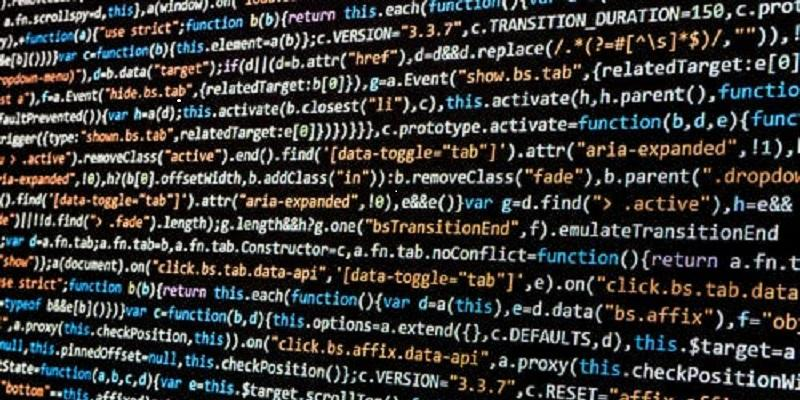# Algorithms and ComplexityTheoretical Computer Science forms the foundations of computing. One of its primary aims is to provide a mathematically grounded theory for developing sophisticated algorithms for real-world problems. This includes mathematical modelling of algorithmic approaches, estimation of the complexity of computational problems, and understanding the limits of efficient computation through computational complexity.

### Our research

The Algorithms and Complexity theme is led by Professor Kristina Vušković. Research within the theme includes graph theory, logic and model theory, combinatorial optimisation, scheduling theory, algorithms on graphs and data structures, the computational complexity of problems on discrete structures, randomized algorithms, probabilistic analysis of algorithms and approximation algorithms.

The research interests of the individual members include:

• Dr Isolde Adler: Structure of graphs and hypergraphs, logic and model theory, (parameterised) complexity theory, database theory, combinatorial games, theory of "big data".
• Professor Martin Dyer: Sampling and counting, randomised algorithms, Markov chains, complexity theory, constraint satisfaction problems, random structures, probabilistic analysis, combinatorial optimisation.
• Professor Raymond Kwan: Public transport scheduling, integer linear programming, heuristics, meta-heuristics, hyper-heuristics. The research has led to Tracsis Plc, a Leeds University spin-out company.
• Dr Haiko Muller: Algorithms on graphs and partially ordered sets, fixed parameter algorithms, approximation algorithms, graph theory, special classes of graphs, width parameters of graphs.
• Dr Natasha Shakhlevich: Deterministic scheduling theory, scheduling with controllable parameters, scheduling algorithms for distributed computing.
• Professor Kristina Vušković: Structural graph theory and graph algorithms.
• Dr Sebastian Ordyniak: Algorithms, complexity, parameterized algorithms, graphs, logic, knowledge representation, mixed integer linear programming, planning.

We also have a large and lively group of postgraduate research students and postdoctoral fellows. We have been very successful in obtaining support from EPSRC and other funders and maintain a high number of international research collaborations (see grants).

### Research seminars

We organise the Algorithms, Logic, and Algebra seminars alongside the School of Mathematics.

Our Logic discussion group is open to researchers and postgraduate students with an interest in logic and its applications.

### Further information

View our research group website.

### PhD projects

We have opportunities for prospective postgraduate researchers. Find out more.

Please contact the group to discuss your own interests. Applicants should note that the research is inherently mathematical, and requires a background and interest in mathematics and/or the mathematical aspects of computer science.